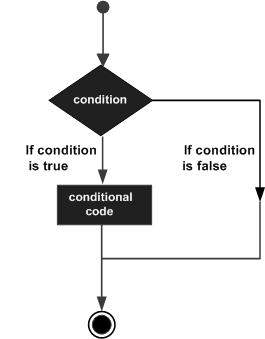# D Programming - Decisions

The decision making structures contain condition to be evaluated along with the two sets of statements to be executed. One set of statements is executed if the condition it true and another set of statements is executed if the condition is false.

The following is the general form of a typical decision making structure found in most of the programming languages −D programming language assumes any non-zero and non-null values as true, and if it is either zero or null, then it is assumed as false value.

D programming language provides the following types of decision making statements.

Sr.No. Statement & Description
1 if statement

An if statement consists of a boolean expression followed by one or more statements.

2 if...else statement

An if statement can be followed by an optional else statement, which executes when the boolean expression is false.

3 nested if statements

You can use one if or else if statement inside another if or else if statement(s).

4 switch statement

A switch statement allows a variable to be tested for equality against a list of values.

5 nested switch statements

You can use one switch statement inside another switch statement(s).

## The ? : Operator in D

We have covered conditional operator ? : in previous chapter which can be used to replace if...else statements. It has the following general form

```Exp1 ? Exp2 : Exp3;
```

Where Exp1, Exp2, and Exp3 are expressions. Notice the use and placement of the colon.

The value of a ? expression is determined as follows −

• Exp1 is evaluated. If it is true, then Exp2 is evaluated and becomes the value of the entire ? expression.

• If Exp1 is false, then Exp3 is evaluated and its value becomes the value of the expression.